﻿ The Effect of Cooperative Learning Type Think Pair Share with Autograph on the Mathematical Representation Ability and Self-efficacyPublications are Open
Access in this journal
Article Versions
Export Article
• Normal Style
• MLA Style
• APA Style
• Chicago Style
Research Article
Open Access Peer-reviewed

### The Effect of Cooperative Learning Type Think Pair Share with Autograph on the Mathematical Representation Ability and Self-efficacy

Yuli Ragelia Sinaga , Edi Syahputra, Faiz Ahyaningsih, Siti Hanijah Br. Saragih
American Journal of Educational Research. 2018, 6(11), 1481-1486. DOI: 10.12691/education-6-11-6
Received October 02, 2018; Revised November 05, 2018; Accepted November 14, 2018

### Abstract

This research attempts the effect of Cooperative Learning type Think Pair Share (TPS) with Autograph on the mathematical representation ability and self-efficacy students. The purpose of this study was to investigate the effect of Cooperative Learning type TPS with Autograph to the students Mathematical Representation Ability and the effect of Cooperative Learning model type TPS with Autograph helping to the students Self-Efficacy. The research samples comprised 36 XI 5 graders (experiment I class) and 36 XI 6 graders (experiment II class) from a Senior High School (SMA) in Medan. We used a quasi experimental design with Cooperative Learning type TPS with Autograph and Cooperative Learning type TPS without Autograph as the independent variables. The dependent variables included the mathematical representation ability and students self-efficacy. The instrument used consisting of the initial mathematical abilities (KAM) test, mathematical representation ability test and self-efficacy questionnaire. The analysis is used two way ANOVA. The research findings indicate that there is a significant effect of Cooperative Learning model type TPS with Autograph to the students mathematical representation ability and there is a significant effect of Cooperative Learning model type TPS with Autograph to the students self-efficacy.

### 1. Introduction

Mathematical standards in schools according to CIAI (Curriculum Instruction Assessment Improvement) Pinellas County Schools include content or material standards (mathematical content), mathematical abilities and process standards (mathematical processes). Standard processes include problem solving, reasoning, communication, connections, and representation. NCTM states that both material standards and process standards together constitute the basic skills and understanding that students desperately need in the 21st century.

From the description above, it can be seen that the ability of mathematical representation is part of the expected abilities in mathematics learning. The ability of representation is very necessary to be presented intensively so that students are actively involved in learning and the loss of the impression that mathematics is a strange and frightening lesson.

According to McCoy, Baker and Little 1, the best way to help students understand mathematics through representation is to encourage them to find or create representations as a means of thinking in communicating mathematical ideas. The use of representation by students can make mathematical ideas more concrete and help students to solve a problem that is considered complex and complex to be simpler if the strategy and utilization of the mathematical representation used is in accordance with the problem. Furthermore, Rusefendi 1 suggested that one of the important roles in learning mathematics is understanding abstract mathematical objects such as facts, concepts, principles and skills. To achieve this requires a presentation of concrete objects to help understand these abstract mathematical ideas, so that in the learning process good representation capabilities are needed. The role of serving concrete objects in learning is limited only as an understanding aid, and if the ideas learned are understood, the presentation of these concrete objects is no longer needed.

Understanding mathematics through representation is by encouraging students to discover and make representations as tools or ways of thinking in communicating mathematical ideas from abstract to concrete. Mathematical representation involves the way students use to communicate how they determine the answer as Hudiono stated 2 that the ability of representation can support students in understanding mathematical concepts learned and their interrelationships; to communicate students' mathematical ideas; to better recognize the connection between mathematical concepts; or applying mathematics to realistic mathematical problems through modeling. Hudiono 2 also states that in Bruner's view, enactive, iconic and symbolic are related to one's mental development, and every higher development of representation is influenced by other representations.

In addition to the importance of mathematical representation abilities, another thing that is considered important is the attitude of students in learning mathematics, one of which is student self-efficacy. Self-efficacy is a person's belief in the ability he has in carrying out and completing tasks so that he can overcome challenges and be able to achieve expected goals. Baron and Byrne 3 define self-efficacy as an evaluation of one's ability or competence in performing a task, to achieve a goal, or to overcome a problem. Confidence in self-efficacy determines how a person feels, thinks, and motivates themselves.

The ability of self-efficacy is not an absolute default. Self-efficacy can be changed, formed, enhanced, derived based on one or a combination of four sources that affect one's self-efficacy, namely: (1) mastery experiences. (2) vicarious experience. (3) verbal persuation, (4) physiological and emotional states. Certain approaches used in learning will be able to improve self-efficacy abilities.

This ability of self-efficacy is also demanded in the mathematics curriculum. The demands of developing the ability of self-efficacy written in the mathematics curriculum, among others, mentions that mathematics must instill an attitude of respecting the usefulness of mathematics in life, which has curiosity, attention, and interest in learning mathematics, as well as resilience and confidence, and problem solving. Mathematical self-efficacy of students develops when they study aspects of mathematical competence. For example, when students develop strategic competencies in solving non-routine problems, many concepts are learned and understood, so that the problem can be solved, in the end the mathematics can be mastered. Conversely, if students are rarely given challenges in the form of mathematical problems to solve, they tend to become memorized rather than follow the proper mathematical learning methods. The example gives rise to two different attitudes. The treatment of the first example will lead to two different attitudes. The first example treatment will lead to an attitude of confidence because students are able to solve mathematical problems. The second treatment will lead to an attitude of easy surrender when faced with a problem, because students are not trained to face challenges. To develop the ability of mathematical representation and self-efficacy students need a mathematical learning approach that is able to foster the ability of mathematical representation and self-efficacy of students.

Learning models that are thought to be able to improve mathematical representation abilities and student self-efficacy are cooperative learning types TPS. TPS cooperative learning is a cooperative learning model that places students in pairs to complete academic tasks through three stages, namely: Think, Pair, and Share. This TPS learning model was developed by Frank Lyman and colleagues from the University of Maryland. This learning model provides opportunities for students to work alone and work with others 4. Furthermore 5 states that TPS cooperative learning can train and develop students 'thinking abilities and activities, because students build knowledge through their own exploration and students' knowledge can also develop through the transfer of thought patterns with other students so students are able to combine and compare mindsets themselves with other students' mindsets. This learning model can stimulate high-level thinking skills because with this learning model the potential possessed by students is really explored as much as possible. In line with that, 6 explains that the advantage of this TPS learning model is to have procedures that are explicitly set to provide more opportunities for students to think, answer, and help each other.

From the expert's opinion, it can be concluded that the TPS cooperative learning model is a learning model that involves the activity of students in learning, because students are told to practice individually, then students are told to work together in pairs and then the results of the discussion are presented in front of the class. And from the expert's opinion, researchers also suspect that this TPS cooperative learning model can improve students' mathematical representation and self-efficacy abilities.

Then in cooperative learning technology takes a very important role to facilitate the learning process. The use of ICT is one of the six principles of the school of mathematics, "Technology is essential in teaching and learning mathematics; it influences the mathematics that is taught and enhances students' learning."For application in class, the use of ICT can be integrated with some learning. As said 7, " There are four different approaches can be implemented in integrating ICT teaching and learning mathematics: (1) Expository learning; (2) Inquiry based learning; (3) Cooperative learning; (4) Individual learning".

There are many mathematical software or software that can be used on computers as ICT-based learning media to make the delivery of mathematics easier, more interesting and students motivated to learn, one of which is the Autograph software. Autograph is software that can be used in mathematics learning especially in two-dimensional and three-dimensional material. At present there is plenty of evidence to show that teaching mathematics at the secondary and tertiary level with dynamic software is far more effective and efficient, and that is especially fun for students and teachers.

Based on the description above, the researcher is interested in conducting a study entitled "The Effect of Cooperative Learning Type Think Pair Share Assisted Autograph on the Ability of Mathematical Representation and Self-Efficacy"

### 2. Methods

Research method uses an experimental method in the form of quasi experimental design. The quasi experimental design method is a design that has a control group, but cannot function fully to control external variables that affect the conduct of experiments.

This research will be conducted in Medan public Senior High School 5. The time for conducting research in the odd semester of the 2017/2018 academic year is for 5 meetings (10 lesson hours = 10 x 45 minutes) for each sample class, with 2 times the test and 3 times face to face.

The population in this study were all students of Medan public Senior High School 5, and the samples in this study were students of class XI. Two classes were selected, one class as the experimental class I and the other as the experimental class II. Experimental class I with the treatment of Cooperative Learning type TPS with Autograph and experimental class II with Cooperative Learning type TPS without Autograph.

The independent variable in this study is the Cooperative Learning type TPS with Autograph and Cooperative Learning type TPS without Autograph. The dependent variable in this study is the ability of mathematical representation and self-efficacy of students after being given treatment.

The research instrument that changed the mathematical representation ability test consisted of 3 essay questions on transformation material and a self-efficacy questionnaire consisting of 30 questions. The test is conducted to determine the extent of improvement in students 'mathematical representation skills before learning through the initial ability test and the ability of students' mathematical representation after learning through the final ability test. The results of the questionnaire were conducted to find out how self-efficacy students 'with different levels of students' mathematical representation abilities.

The research design used in this study is the design of non-equivalent control groups with factorial 3 x 2 analysis designs. In this experimental design there are initial abilities, different treatments, and final abilities. The design of the non-equivalent control group according to 8 is shown in Table 1 as follows:

After the test, the data analysis will then be carried out in the form of homogeneity test using the F test, normality test using kolmogorov-smirnov and hypothesis testing. Calculation of the results of the study using Excel 2010 and SPSS 19.

The statistical hypothesis test used is two-way Anova using SPSS version 19.0. The statistical model for this experiment is: 9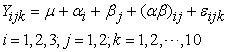(1)

Description:= the score of the students' mathematical representation ability, in the first KAM, which gets the learning j.

μ= the average score of the actual mathematical representation ability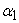= additive effect of the i KAM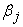= additive effect of the j learning model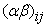= the effect of the interaction of the first KAM and the jth learning model= the effect of experimental deviation from the student score k, in the first KAM, which gets the learning j.

Assumptions that must be met and vice versa are tested statistically, namely:

1. All components in the right hand side of the linear model equation above are additive.

2. The influence of KAM, the learning model and the interaction between KAM and the learning model are constant.

3. Experimental deviations are normally distributed, with averages = 0 and variances σ2.

### 3. Results and Discussion

3.1. Description of Students' Mathematical Representation Ability

Representation ability tests are the final test or post test in this study. Tests of representation ability in the form of essay questions related to the material being experimented on are material transformation. Questions consisting of 3 questions represent 3 (three) indicators of representation ability, namely: Presenting data or information from a representation to a representation of diagrams, graphs or tables (indicator 1), finishing the problems by involving mathematical expressions (indicator 2), and answering the questions using written words or texts (indicator 3). The percentage results of a summary of the achievements of the experimental students can be seen in the following diagram:

• Figure 1. Mathematical Representation Ability Diagram in Experimental Class I

Based on Figure 1, it can be concluded that the highest indicator mastered by students is indicator 2, which is equation or mathematical expression.

Based on Figure 2, it can be concluded that the highest indicator mastered by students is indicator 1 which is presenting the data in the form of diagrams, tables, images or graphs.

• Figure 2. Mathematical Representation Ability Diagram in Experimental Class II
3.2. Student Mathematical Representation and Self-Efficacy Questionnaire Test Results

This data was obtained from the results of test scores on mathematical representation abilities and self-efficacy questionnaires from experimental class I and experimental class II.

In Table 2 above can be seen the average representation ability and self-efficacy of the two groups of students learned by cooperative learning type TPS assisted by Autograph and TPS type cooperative learning without the help of Autograph.

3.2.1. Data Normality Test Ability of Mathematical Representation and Self-efficacy

The formulation of hypotheses to test the normality of data is:

H0: samples come from populations that are normally distributed

H1: samples come from populations that are not normally distributed.

Testing criteria used is if the value of significance (sig.) Is greater than α = 0.05 then H0 is accepted. Data normality test used the Kolmogorov-Smirnov test.

From Table 3 the results give a significance value greater than the significance value (sig). This means that the scores of mathematical representation ability of students from both sample groups have normal variance.

From Table 4, the experimental class I and experimental class II self-efficacy data have a significance value greater than 0.05, that is (0.373 > 0.05) and (0.100> 0.05) then the self-efficacy data for cooperative learning type TPS assisted by Autograph and TPS type cooperative learning without the help of Autograph are normally distributed.

3.2.2. Data Homogeneity Test Ability of Mathematical Representation and Self-efficacy

The formulation of the statistical hypothesis to test the homogeneity of variance between the two groups of data is:

H0: σ12 = σ22:both samples come from populations that have homogeneous variances

H1: σ12 ≠ σ22:two samples come from populations that have variances that are not homogeneous

Test criteria used is if the value of significance (sig.) is greater than α = 0.05, H0 accepted.

Based on Table 5 gives the significance value (sig.) = 0.930 greater than α = 0.05, H0 accepted. Similarly in Table 6 value (sig.) = 0.580 greater than α = 0.05, H0 accepted. Thus both samples come from populations that have a homogeneous variance.

3.2.3. Hypothesis Test

Hypothesis I: Test the first research hypothesis which says whether there is a significant effect of Autograph-assisted TPS type cooperative learning on students' mathematical representation abilities.

Statistically the hypothesis can be formulated: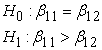Description:: the effect of cooperative learning type TPS assisted on students 'mathematical representation ability: the effect of cooperative learning type TPS without Autograph assistance on students' mathematical representation ability

Based on the two way ANOVA in Table 7 above, the p-value was obtained for the study was 0.002 < 0.05, that is enough evidence to reject H0 and accept H1. It means that there is the influence of Autograph-assisted TPS type cooperative learning on students' mathematical representation abilities.

Hypothesis II: Test the second research hypothesis which says whether there is a significant effect of TPS-type cooperative learning assisted by Autograph on student self-efficacy.

Statistically the hypothesis can be formulated: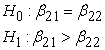Description: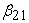: the effect of Autograph type assisted TPS cooperative learning on students' mathematical representation ability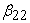: the effect of TPS type cooperative learning without Autograph assistance on students' mathematical representation ability

Based on the two way ANOVA in Table 8 above, the p-value was obtained for the study was 0.048 < 0.05, that is enough evidence to reject H0 and accept H1. It means that there is the effect of TPS-assisted cooperative learning on student self-efficacy.

3.3. Discussion of Research

The description of several factors related to this study are as follows:

3.3.1. Mathematical Representation Ability

From the results of calculations, it was found that there was the effect of Autograph type assisted TPS learning on students' mathematical representation abilities. In other words the effect of cooperative learning type TPS assisted on the ability of mathematical representation is better than TPS type cooperative learning without Autograph assistance on mathematical representation abilities. Students who take part in learning with assisted TPS type cooperative learning Autograph have been accustomed to being active in solving problems thinking individually to get concepts. Because the learning process is not just transferring knowledge from the teacher to students, but rather a process that is conditioned or sought by the teacher, so students are active in various ways to build their own knowledge. In line with Piaget which emphasizes the importance of student motivation and facilitation by the teacher. In order for children's intellectual development to take place optimally, they need to be motivated and facilitated to develop theories that explain the world around them.

In line with view 10 says that through representation, difficult problems can be seen more easily and simply, so that the problems given can be solved easily. According to 11 he stated that the same thing is that students who are able to apply the ability of representation will be able to more easily solve problems and more easily understand them. According to 12 the ability of representation has a very important role in learning mathematics that every student needs to have. This means that in solving mathematical problems the ability of representation has an important role so students can express their mathematical ideas in the problem solving process.

3.3.2. Students Self-efficacy

Self-efficacy is a student's confidence / self-confidence that is measured by one's ability to think of strategies in facing difficulties, strategies to avoid problems that are beyond their ability, ability to solve different problems, beliefs with self-abilities and not easy to despair. Reference 13 states "Self-efficacy as a means of beliefs about their ability to produce their level of performance that is indicated by their beliefs about their ability to produce lives." influence on events that affect their lives. This study uses indicators from sources that influence the level (Level) self-efficacy. generality and strength. Self-efficacy is as a person's beliefs about their ability to produce a level of performance.

Research conducted by 14 concluded that the self-efficacy of students in Muhammadiyah SMAS 8 Kisaran after learning using Jigsaw model cooperative learning was getting better. This can be seen from 34 students in learning, 26.47% have high self-efficacy, 61.76% students have moderate self-efficacy, and 11.76% students have low self-efficacy. In general, 73.31% of students are at moderate level self-efficacy. In addition, the research conducted 15 concluded that the increase in self-efficacy students can improve student learning outcomes.

From the results of calculations, it was found that there was the effect of TPS-assisted cooperative learning on student self-efficacy. In other words, the influence of Autographed Assisted Type TPS cooperative learning on student self-efficacy is better than TPS type cooperative learning without Autograph help on student self-efficacy.

### 4. Conclusions

Based on the results of the analysis and discussion in this study, several conclusions are presented as follows:

1. There is the effect of cooperative learning type TPS by Autograph on the ability of mathematical representation, when viewed from the difference in the average cooperative learning TPS assisted Autograph with cooperative learning TPS type without Autograph help in influencing the results of mathematical representation ability while still assuming other uncontrolled factors in influencing mathematical representation ability.

2. There is the effect of cooperative type TPS on the ability of self-efficacy, if seen from the difference in the average cooperative type TPS with the help of cooperative type TPS with Autograph in influencing the results of self-efficacy ability while still considering other factors that are uncontrollable in influencing self-efficacy.

### Acknowledgments

On this occasion the author expresses his deepest gratitude to my beloved Father and Mother (Mr. A. Sinaga and Mrs. Ponimi), brothers (Evan and Muklis), and sister (Sry Adelina) and all my beloved families who always pray for, give full support and motivation in completing this paper.

### References

  Hutagaol, K, “Contextual Learning to Enhance the Mathematical Representation Ability of Middle School Students,” Jurnal Infinity, Bandung, Universitas Advent Indonesia, 2013. In article  Nursangaji, A., Aryanti, D., dan Zubaidah, “Mathematical Representation Ability According to Ability Levels Students in Quadrilateral Material in Middle School,” Jurnal Untan, 2013. In article  Fitriani, N., Subekti, A. dan Aquarisnawati, P, “The Effect of Self-efficacy Emotional Maturity on Craving in Former Drug Users,” Journal INSAN, Vol 3, Universitas Hang Tuah Surabaya, 2011. In article  Isjoni, H, Cooperative Learning Enhances Communication Intelligence among Students, Pustaka Pelajar, Pekanbaru, 2009. In article  Trianto, Models of Constructivist Oriented Learning, Prestasi Pustaka, Jakarta, 2007. In article  Nurhadi, Contextual Learning, JP Books, Surabaya, 2009. In article  Karnasih, I, “ICT for teaching and Learning Mathematics”, In Collaboration between UNIMED and QED Education Kuala Lumpur, Malaysia, 2008. In article  Sugiyono, Quantitative, Qualitative, and R & D Research Methods, Alfabeta, Bandung, 2013. In article  Syahputra, E, Applied Statistics for Quasi and Pure Experiments in Education, Biology, Agriculture, Engineering, etc. UNIMED PRESS, Medan, 2016. In article PubMed  PubMed  Istiawati, Mulyono, dan Syahputra, E, “The Effect of Based Learning Problem Model with Macromedia Flash to the Representation Mathematical Ability VII Grade Students of SMPN 1 Sidamanik”, Journal of Education and Practice, Vol.8, No.23, ISSN 2222-1735, 2017. In article  Sajadi, M, Amiripour, P, dan Malkhalifeh, M. R, “The Examining Mathematical Word Problems Solving Ability under Efficient Representation Aspect”, International Scientific Publications and Consulting Service, Vol. 2013, 2013. In article  Syahputra, E dan Marpaung, R.I.T. “Students’ Mathematics Representation and The Alternative Solutions”, Proceedings of the 1st AISTEEL, Vol. 1,2016 e-ISSN 2548-4613, 2016. In article  Bandura, A. “Self-Efficacy”, In V. S. Ramachaudran (Ed.), Encyclopedia of human behavior, Academic Press, New York, Vol. 4, pp. 1-14, 1997. In article  Sari, D.P, Syahputra, E dan Surya, E, “An Analysis of Spatial Ability and Self-efficacy of Students in Cooperative Learning by Using Jigsaw at SMAS Muhammadiyah 8 Kisaran” American Journal of Educational Research. Vol. 6, No. 8, 1238-1244, 2018. In article View Article  Sapta, A, Syahputra, E dan Hamid, A. “The Use of Rewards in Improving Self-Efficacy”, 2nd AISTEEL. volume 104, 2017. In article

Published with license by Science and Education Publishing, Copyright © 2018 Yuli Ragelia Sinaga, Edi Syahputra, Faiz Ahyaningsih and Siti Hanijah Br. SaragihThis work is licensed under a Creative Commons Attribution 4.0 International License. To view a copy of this license, visit http://creativecommons.org/licenses/by/4.0/